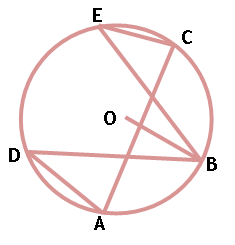Maths / Circles / Angles in the Same Segment are Equal

QUESTION

 If A and E points are joined, which of the following relations is true ?​OPTIONS A. B. C. D.
Right Option : C

EXPLANATION
Explain TypeExplanation Content
Text

When AE is joined then

because angles in the same segment are equal

TestimonialsSTUDENT RESPONSE - Barkha Arora C/o ABHYAS Academy
10th
It has a great methodology. Students here can get analysis to their test quickly.We can learn easily through PPTs and the testing methods are good. We know that where we have to practice